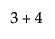Scripting Guide > Common Tasks > Convert Character Dates to Numeric Dates
Publication date: 08/13/2020

# Convert Character Dates to Numeric Dates

Data might appear to be numeric in the data table. However, the column properties may specify a character data type. To manipulate the data as date/time values, convert the column to a numeric column and specify how you want the values to appear.

Convert Dates.jsl creates a data table, specifies the data input format, changes the column to a numeric continuous column, and applies the m/d/y format (Figure 17.1).

// Create a data table with character dates.

`dt = New Table( "Example",`
`	Add Rows( 3 ),`
`	New Column( "Dates",`
`		Character,`
`		Nominal,`
`		Set Values( {"25/01/2010", "30/09/2009", "15/12/2013"} )`
`	)`
`);`
` `

// Display a modal dialog for the user to confirm the format conversion.

`nw = New Window( "Date Conversion",`
`	<<Modal,`
`	tb = Text Box(`
`		"Notice the d/m/y format of the character dates.`
`Click OK to convert the column to a numeric column and apply the m/d/y format."`
`	)`
`);`
` `

/* Apply the Numeric data type.

Specify the Informat (input format) value "d/m/y".

Specify the Format (display format) value "m/d/y".

Apply the Continuous modeling type */

`col = Column( dt, "Dates" );`
`col << Data Type( "Numeric", Informat( "d/m/y" ), Format( "m/d/y" ) );`
`col << Modeling Type( "Continuous" );`

Figure 17.1 Converting Character Dates (Before and After)When you change the column’s data type from character to numeric, defining the format in which the data were entered is important. In this example, Informat( "d/m/y" ) defines the input format. Format( "m/d/y" ) defines the new display format. If Informat() is omitted, the Format() value is applied as both the input and display format. This results in missing values for some data.

Modify Convert Dates.jsl to see for yourself.

1. Open Convert Dates.jsl from the sample scripts folder.

2. Right-click the script window and select Show Line Numbers.

3. On line 9, change "25/01/2010" to "01/25/2010".

4. On line 27, delete Informat( "d/m/y" ), (including the comma).

5. Run the script.

Format( "m/d/y" ) is applied to the column. Only "01/25/2010" appears in the column. The other values are missing; "30/09/2009" and "15/12/2013" are not valid m/d/y values.